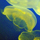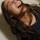## General Question# Math geeks: How do i do eigenvalue decomposition of a scalar matrix?

Asked by nisse (1986) March 17th, 2010

I know how to find eigenvalues of a normal full-rank matrix, but how do i find the eigenvalues if the matrix is already scalar to begin with? I know i want to do det(A-I*lambda)=0, but this only gives me one of the eigenvalues, how do i find the second one?

For example how to calculate eigs(A), where A=[0.7 0; 0 0.7].

I know lambda_1 is = 0.7, and that lambda_2 is supposed to be = 1, but i dont understand why. Are the repeated eigenvalues of a scalar matrix always 1? Are the eigenvalues of B=[0.7 0 0; 0 0.7 0; 0 0 0.7] = [0.7;1;1] and if so why?

Observing members: 0Composing members: 0## 3 AnswersYour information isn’t quite accurate. Finding eigenvalues of scalar matrices is pretty much the easiest thing in the world: they are the scalar value with multiplicity n (where n is the size of the matrix). In your example, the eigenvalues are 0.7 with multiplicity 2 (because it shows up twice).

In general, you can easily read the eigenvalues from any triangular matrix (which includes scalar matrices by definition) just by taking the values on the diagonal.

You might be getting at something more nuanced than this, though, so please feel free to refine your question and I’ll try my best to help. I used to teach this, and really love linear algebra. This is fun for me. :)

elenuial (1405)“Great Answer” (3) Flag as…That’s was actually my first impulse aswell (0.7 with multiplicity 2), my problem had a typo which had made me uncertain. I figured there was something peculiar about scalar matrices and could hardly find anything when trying to google it.

There was nothing more to it :) Big thanks.

nisse (1986)“Great Answer” (1) Flag as…Response moderated (Spam)

## Answer this questionor

#### Join

to answer.

This question is in the General Section. Responses must be helpful and on-topic.

Your answer will be saved while you login or join.
Have a question? Ask Fluther!
What do you know more about?
or
Knowledge Networking @ Fluther